## IAC-STAR

### SYNTHETIC COLOR-MAGNITUDE DIAGRAM COMPUTATION ALGORITHM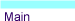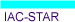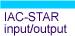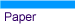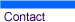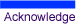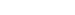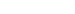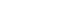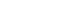A. Your Personal Data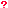Name Institution e-mail

 B. LibrariesSelect stellar evolution library Bertelli 94 Teramo Girardi00 Select bolometric correction library Lejeune et al. 1997 Castelli & Kurucz 2002 Girardi et al. 2002 Origlia & Leitherer 2000 (HST-WFPC2) Bedin et al. 2005 (HST-ACS)

 C. Control ParametersTotal number of  computed  stars (n) Number of stars saved to output file (m) Limiting magnitude for stars to be written in the ouput file Filter <

 C-2. Mass loss at RGB and AGBMass loss parameter at the RGB Mass loss parameter at the AGB

 D. Star Formation RateTwo formats are possible 1. Provinding n sampling points: n, t[1->n], SFR[1->n],  (max n=20; t(n) is the present age (in Gyr) of the system) 2. Exponencial law of the form SFR=exp(t/-β): 1, T, β (T is the present age (in Gyr)) 2, 0, 13, 1, 1

 E. Metallicity LawE.1) Defined by sampling points
 Lower or unique law Upper law (optional) n, t[1->n],  Z[1->n] (max n=20, 0 to define law by physical parameters: see below) n, t[1->n],  Z[1->n] (max n=20, 0 to ignore)

E.2) Defined by physical parameters (all parameters 0 to ignore)

 Lower or unique law Upper law (optional) Starting Z (Zi) Starting Z (Zi) Final Z (Zf ) Final gas fraction (μf) Final gas fraction (μf) Infall parameter (α) Infall parameter (α) Outflow parameter (λ) Outflow paramter (λ)

 F. Initial Mass FunctionIMF: n, m [1->n], x [1->(n-1)] 3, 0.1, 0.5, 120., -1.3, -2.3

 G. Binary StarsFraction of binary stars (f) Minimum mass ratio (q)

 H. Random Number GeneratorSeed

Note: If you receive an "internal server error" message, it means that the computation time (which depend on the input parameters) has exceeded a server-dependent time limit. However, this does not affect the iac-star job itself, which will complete and, when finished, send you an email with the download link.